# Amazing progress in adversarially robust stochastic multi-armed bandits

In this post I briefly discuss some recent stunning progress on robust bandits (for more background on bandits see these two posts, part 1 and part 2, in particular what is described below gives a solution to Open Problem 3 at the end of part 2).

In multi-armed bandit problems the gold standard property, going back to a seminal paper of Lai and Robbins in 1985 is to have a regret upper bounded by:

(1)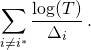Let me unpack this a bit: this is for the scenario where the reward process for each action is simply an i.i.d. sequence from some fixed distribution,is the index of the (unique) best action, and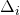is the gap between the mean value of the best action and the one of. Such guarantee is extremely strong, as in particular it means that actions whose average performance is a constant away from the optimal arm are very rarely played (only of order). On the other hand, the price to pay for such an aggressive behavior (by this I mean focusing on good actions very quickly) is that all the classical algorithms attaining the above bound are extremely sensitive to adversarial examples: that is if there is some deviation from the i.i.d. assumption (even very brief in time), the algorithms can suddenly suffer linear inregret.

Of course there is an entirely different line of works, on adversarial multi-armed bandits, where the whole point is to prove regret guarantee for any reward process. In this case the best one can hope for is a regret of order. The classical algorithm in this model, Exp3, attains a regret of order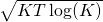. In joint work with Jean-Yves Audibert we showed back in 2009 that the following strategy, which we called PolyINF, attains the optimal: view Exp3 as Mirror Descent with the (negative) entropy as a regularizer, and now replace the entropy by a simple rational function namelywith(this mirror descent view was actually derived in a later paper with Gabor Lugosi). The proof becomes one line (given the appropriate knowledge of mirror descent and estimation in bandit games): the radius part of the bound is of the form, while the variance is of the form (since the inverse of the Hessian of the mirror map is a diagonal matrix with entries):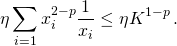Thus optimizing overyieldsfor any. Interestingly, the best numerical constant in the bound is obtained for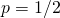.

Best of both worlds

This was the state of affairs back in 2011, when with Alex Slivkins we started working on a best of both worlds type algorithm (which in today’s language is exactly a stochastic MAB robust to adversarial examples): namely one that gets theguarantee (in factin our original paper) if the environment is the nice i.i.d. one, and also(in fact) in the worst case. This original best of both worlds algorithm was of the following form: be aggressive as if it was a stochastic environment, but still sample sufficiently often the bad actions to make sure there isn’t an adversary trying to hide some non-stochastic behavior on these seemingly bad performing actions. Of course the whole difficulty was to show that it is possible to implement such a defense without hurting the stochastic performance too much (remember that bad actions can only be sampled of ordertimes!). Since this COLT 2012 paper there has been many improvements to the original strategy, as well as many variants/refinements (one such variant worth mentioning are the works trying to do a smooth transition between the stochastic and adversarial models, see e.g. here and here).

A stunning twist

The amazing development that I want to talk about in this post is the following: about a year ago Julian Zimmert and Yevgeny Seldin proved that the 2009 PolyINF (crucially with) strategy actually gets the 2012 best of both worlds bound! This is truly surprising, as in principle mirror descent does not “know” anything about stochastic environments, it does not make any sophisticated concentration reasoning (say as in Lai and Robbins), yet it seems to automatically and optimally pick up on the regularity in the data. This is really amazing to me, and of course also a total surprise that the polynomial regularizer has such strong adaptivity property, while it was merely introduced to remove a benign log term.

The crucial observation of Zimmert and Seldin is that a a certain self-bounding property of the regret implies (in a one-line calculation) the best of both worlds result:

Lemma 1: Consider a strategy whose regret with respect to the optimal actionis upper bounded by

(2)(Recall that for multi-armed bandit one selects a probability distributionover the actions, sodenote here the probability of playing actionat time.) Then one has that the regret is in fact bounded by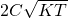(this follows trivially by Jensen on thesum), and moreover if the environment is stochastic one has that the regret is in fact bounded bytimes.

Proof: Assuming that the environment is stochastic we can write the regret as, so by assumption and using thatone has:which means that the left hand side is smaller thanwhich is indeed smaller thantimes.

Yet another one-line proof (okay, maybe 5 lines)

Zimmert and Seldin proved that PolyINF withactually satisfies the self-bounding property of Lemma 1 (and thus obtains the best of both worlds guarantee). In another recent paper by Zimmert, in joint work with Haipeng Luo and Chen-Yu Wei, they simplify the analysis by using a very mild variation of the PolyINF regularizer, namely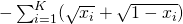. In my view it’s the proof from the book for the best of both worlds result (or very close to it)! Here it is:

Lemma 2: Equation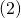withis an upper bound on the regret of mirror descent with learning, mirror map, and standard multi-armed bandit loss estimator.

Proof: The classical mirror descent analysis from any good book will tell you that the regret is controlled by (for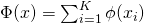and with the convention):

(3)We now consider those terms for the specificandsuggested in the lemma. First notice that. Moreover(sinceis integral) so that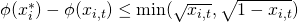. In other words the first term inis upper bounded by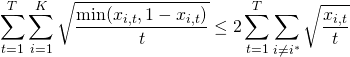where the inequality simply comes from.

Next note that, so that the second term inis upper bounded by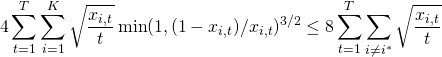where the inequality follows trivially by considering the two cases whetheris smaller or larger than.

This entry was posted in Machine learning, Optimization, Theoretical Computer Science. Bookmark the permalink.

## 6 Responses to "Amazing progress in adversarially robust stochastic multi-armed bandits"

••••••